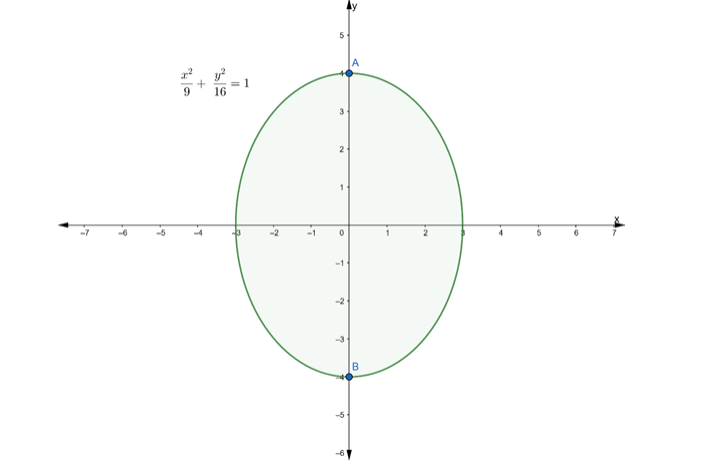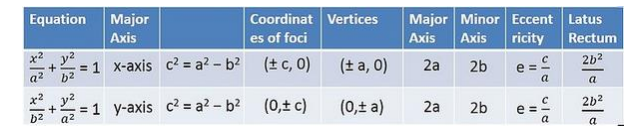Deepak Scored 45->99%ile with Bounce Back Crack Course. You can do it too!

# Find the value

Question:

Find the

(i) lengths of major axes,

(ii) coordinates of the vertices,

(iii) coordinates of the foci,

(iv) eccentricity, and

(v) length of the latus rectum of each of the following ellipses.

$\frac{x^{2}}{9}+\frac{y^{2}}{16}=1$

Solution:Given:

$\frac{x^{2}}{9}+\frac{y^{2}}{16}=1$ ..........(i)Since, $9<16$

So, above equation is of the form,

$\frac{x^{2}}{b^{2}}+\frac{y^{2}}{a^{2}}=1$ ...........(ii)

Comparing eq. (i) and (ii), we get

$a^{2}=16$ and $b^{2}=9$

$\Rightarrow a=\sqrt{16}$ and $b=\sqrt{9}$

$\Rightarrow a=4$ and $b=3$

(i) To find: Length of major axes

Clearly, $a$\therefore$Length of major axes$=2 \mathrm{a}=2 \times 4=8$units (ii) To find: Coordinates of the Vertices Clearly,$a>b\therefore$Coordinate of vertices$=(0, a)$and$(0,-a)=(0,4)$and$(0,-4)$(iii) To find: Coordinates of the foci We know that, Coordinates of foci$=(0, \pm c)$where$c^{2}=a^{2}-b^{2}$So, firstly we find the value of$cc^{2}=a^{2}-b^{2}=16-9c^{2}=7c=\sqrt{7} \ldots(l)\therefore$Coordinates of foci$=(0, \pm \sqrt{7})$(iv) To find: Eccentricity We know that, Eccentricity$=\frac{\mathrm{c}}{\mathrm{a}}\Rightarrow \mathrm{e}=\frac{\sqrt{7}}{4}[$from$(\mathrm{I})]$(v) To find: Length of the Latus Rectum We know that, Length of Latus Rectum$=\frac{2 b^{2}}{a}=\frac{2 \times(3)^{2}}{4}=\frac{9}{2}\$